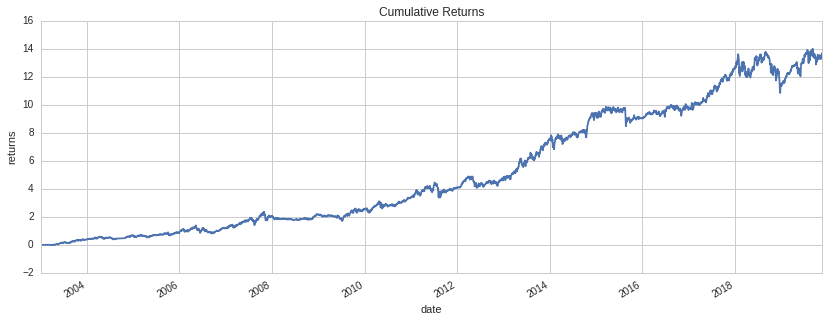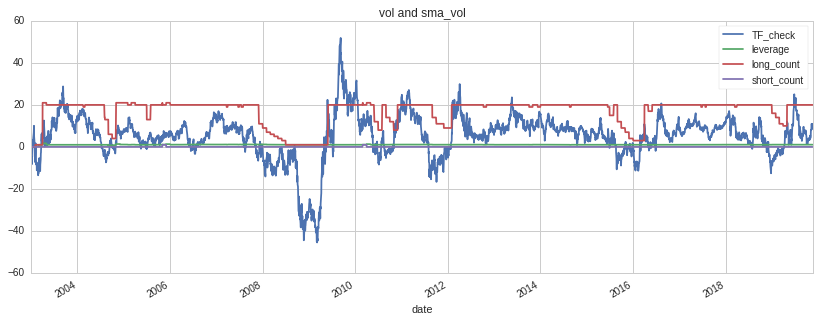Notebook

## Quality Companies in an Uptrend (original by Chris Cane) Long-Short Count and TF Check¶

In :
import pyfolio as pf
import matplotlib.pyplot as plt
import empyrical  as ep

In :
cum_returns = ep.cum_returns(returns)
ax = cum_returns.plot(figsize=(14,5))
ax.set(title='Cumulative Returns', ylabel='returns', xlabel='date');In :
ax = bt.recorded_vars.plot(figsize=(14,5))
ax.set(title='Long-Short Count and TF Check', xlabel='date');In [ ]: